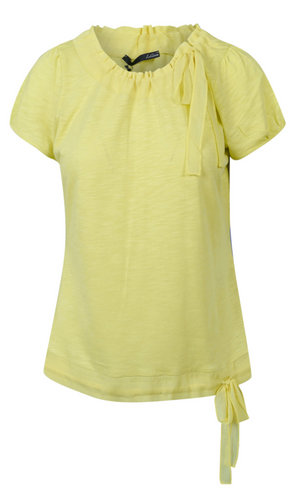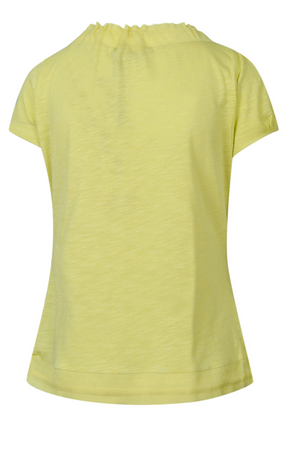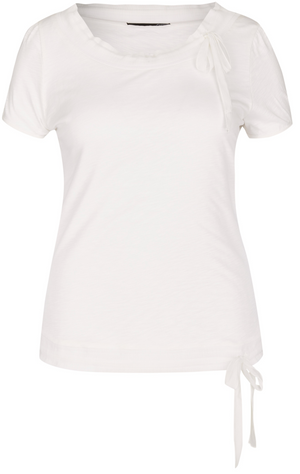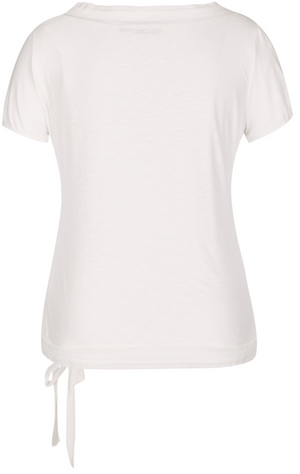le comte

# Drawstring Tee

Regular price \$115 now \$57.50 Unit price  per

Shipping calculated at checkout.
• 50% cotton 50% viscose
• Machine wash cold
• Hang to dry
• Side drawstring on waist & collar
• Short sleeve
• Fits true to size

Size Guide

• 34 = 4 = XS
• 36 = 6 = S
• 38 = 8 = M
• 40 = 10 = M
• 42 = 12 = L
• 44 = 14 = L
• 46 = 16 = XL